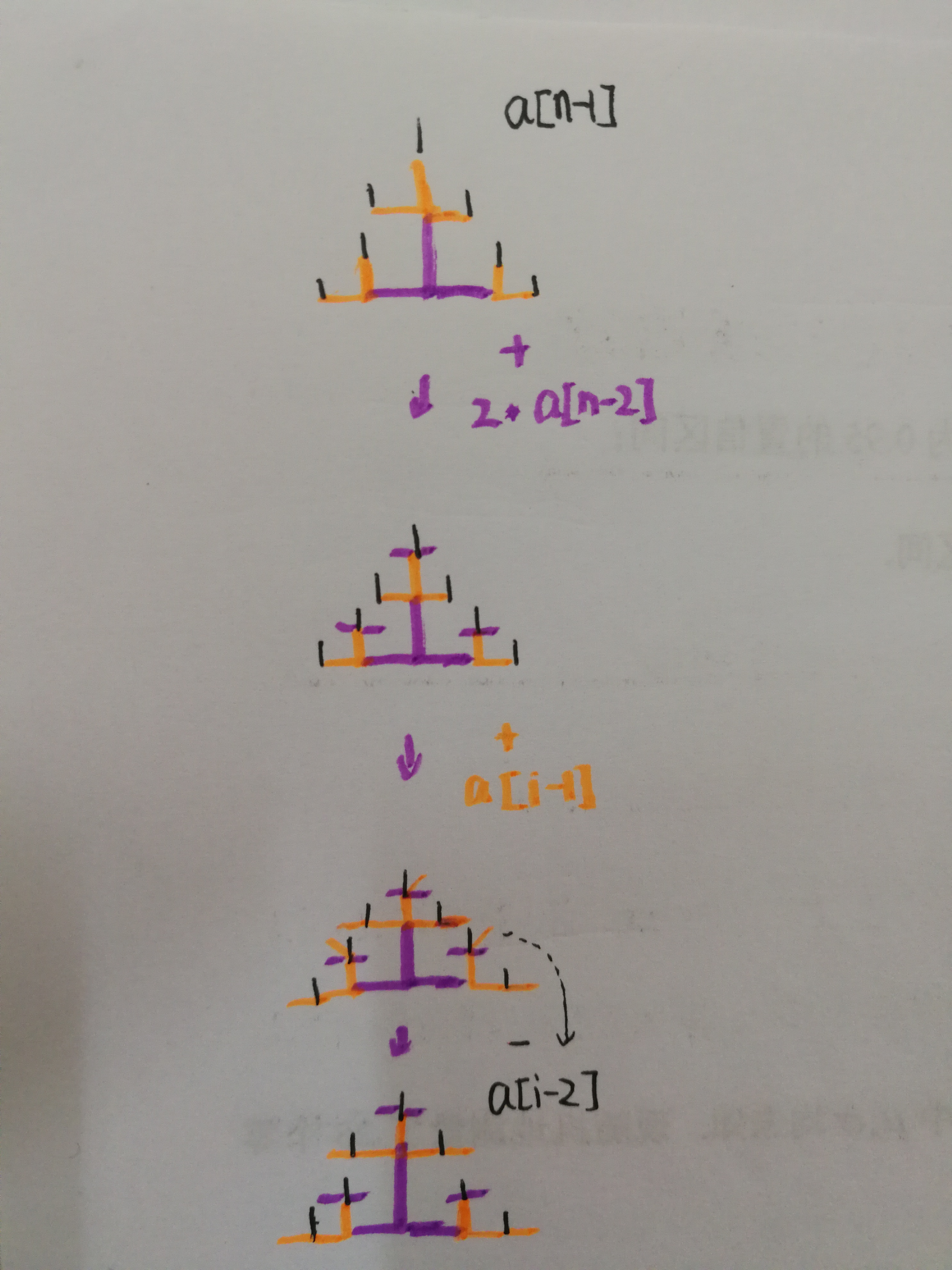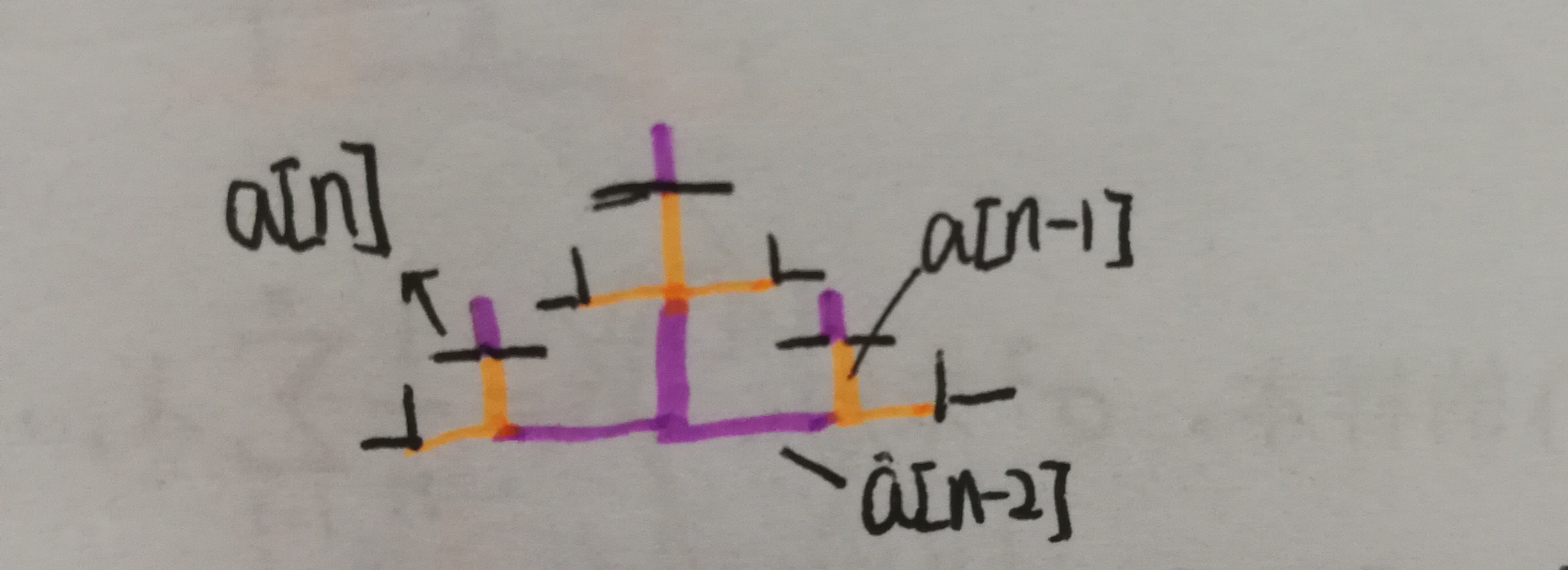# 递推 踩方格问题 思路分析

a. 每走一步时，只能从当前方格移动一格，走到某个相邻的方格上；
b. 走过的格子立即塌陷无法再走第二次；
c. 只能向北、东、西三个方向走；

a[n] = a[n-1] + a[n-1] + a[n-1];

a[n] = a[n-1] + a[n-1];

a[n] = a[n-1] + a[n-1];

a数组存的是向上的步数，a, a数组分别存的是向左和向右的步数，那么最后就是结果就是a[n] + a[n] + a[n].当时做出来还不太在意，看别的题的答案时顺带看了眼题解，哦！？f[n] = 2f[n-1]+f[n-2]???这式子怎么来的？？然后再次开始思考这道本质，或者说是这个式子的由来。f[n] = a[n] + a[n] + a[n];

a[n] = a[n-1] + a[n-1] + a[n-1];

a[n] = a[n-1] + a[n-1];

a[n] = a[n-1] + a[n-1];

f[n] = 3 * a[n-1] + 2 * a[n-1] + 2 * a[n-1] = 2 * f[n-1] + a[n-1]

04-12641
04-2321804-03164
12-02528
10-2164
05-10627
04-17676
02-10229
04-17170